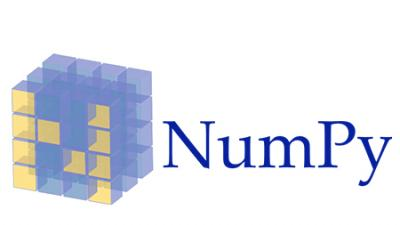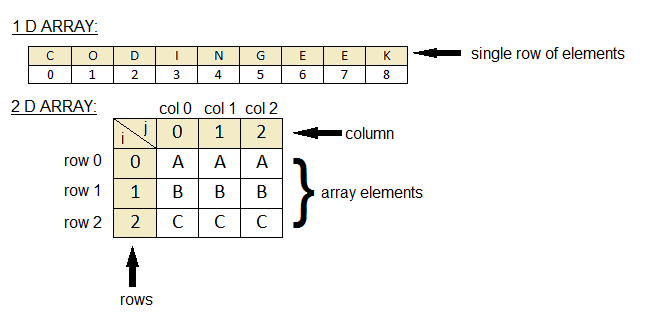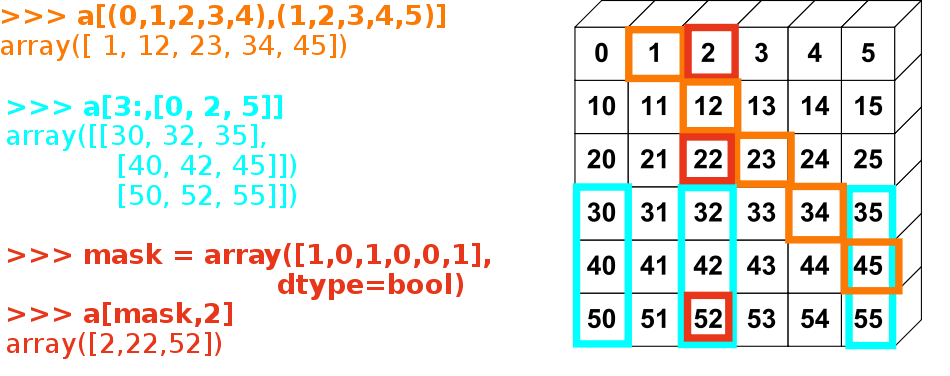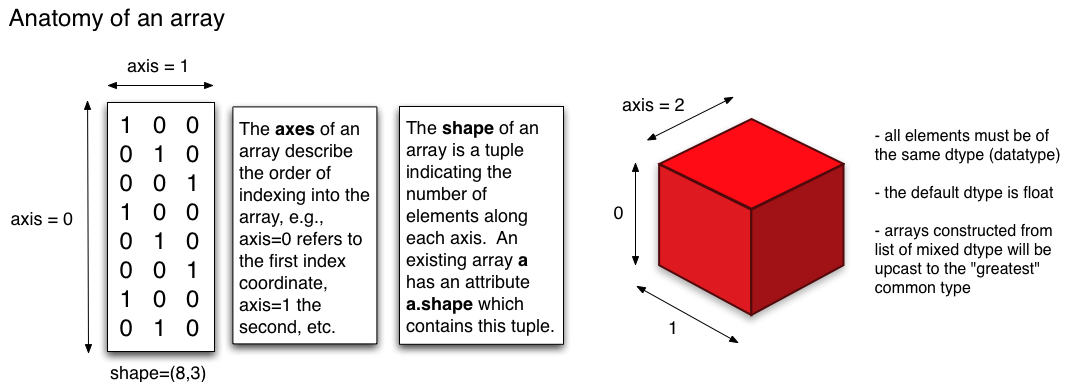# 從零開始學資料科學：Numpy 基礎入門# 什麼是 Numpy？

Numpy 是 Python 的一個重要模組（Python 是一個高階語言也是一種膠水語言，可以透過整合其他低階語言同時擁有效能和高效率的開發），主要用於資料處理上。Numpy 底層以 C 和 Fortran 語言實作，所以能快速操作多重維度的陣列。當 Python 處理龐大資料時，其原生 list 效能表現並不理想（但可以動態存異質資料），而 Numpy 具備平行處理的能力，可以將操作動作一次套用在大型陣列上。此外 Python 其餘重量級的資料科學相關套件（例如：Pandas、SciPy、Scikit-learn 等）都幾乎是奠基在 Numpy 的基礎上。因此學會 Numpy 對於往後學習其他資料科學相關套件打好堅實的基礎。

# Numpy 基礎操作1. Numpy 陣列
Numpy 的重點在於陣列的操作，其所有功能特色都建築在同質且多重維度的 ndarray（N-dimensional array）上。ndarray 的關鍵屬性是維度（ndim）、形狀（shape）和數值類型（dtype）。 一般我們稱一維陣列為 vector 而二維陣列為 matrix。一開始我們會引入 numpy 模組，透過傳入 listnumpy.array() 創建陣列。

 # 引入 numpy 模組
import numpy as np
np1 = np.array([1, 2, 3])
np2 = np.array([3, 4, 5])

# 陣列相加
print(np1 + np2) # [4 6 8]

# 顯示相關資訊
print(np1.ndim, np1.shape, np1.dtype) # 1 (3,) int64 => 一維陣列, 三個元素, 資料型別

np3 = np.array([1, 2, 3, 4, 5, 6])


從檔案取資料：

 npd = np.genfromtxt('data.csv', delimiter=',')


改變陣列維度：

 np3 = np3.reshape([2, 3])
print(np3.ndim, np3.shape, np3.dtype) # 2 (2, 3) int64


改變陣列型別（bool、int、float、string）：

bool 可以包含 True、False，int 可以包含 int16、int32、int64。其中數字是指 bitsfloat 可以包含 16、32、64 表示小數點後幾位。string 可以是 string、unicode。nan 則表示遺失值。

 np3 = np3.astype('int64')
np3.dtype
# dtype('int64')

2. 建立陣列
建立填滿 0 或 1 的陣列：

 np1 = np.zeros([2, 3]) # array([[ 0.,  0.,  0.], [ 0.,  0.,  0.]])
np2 = np.ones([2, 3]) # array([[ 1.,  1.,  1.], [ 1.,  1.,  1.]])1. 陣列索引與切片
一維陣列操作和 Python 原生 list 類似：

 np3 = np.array([1, 2, 3, 4, 5, 6])
print(np3) # 3


二維陣列：

 np3 = np3.reshape([2, 3])

print(np3[1, 0]) # 41. 基本操作
使用布林遮罩來取值：

 np3 = np.array([1, 2, 3, 4, 5, 6])
print(np3 > 3) # [False False False  True  True  True]
print(np3[np3 > 3]) # [4 5 6]


加總：

 np3 = np3.reshape([2, 3])
print(np3.sum(axis=1)) # 將 axis=1 橫向加總 [6 15]


# 延伸閱讀

（image via berkeleycodingeekcornellscipy-lectures

@kdchang 文藝型開發者，夢想是做出人們想用的產品和辦一所心目中理想的學校。A Starter & Maker. JavaScript, Python & Arduino/Android lover.:)